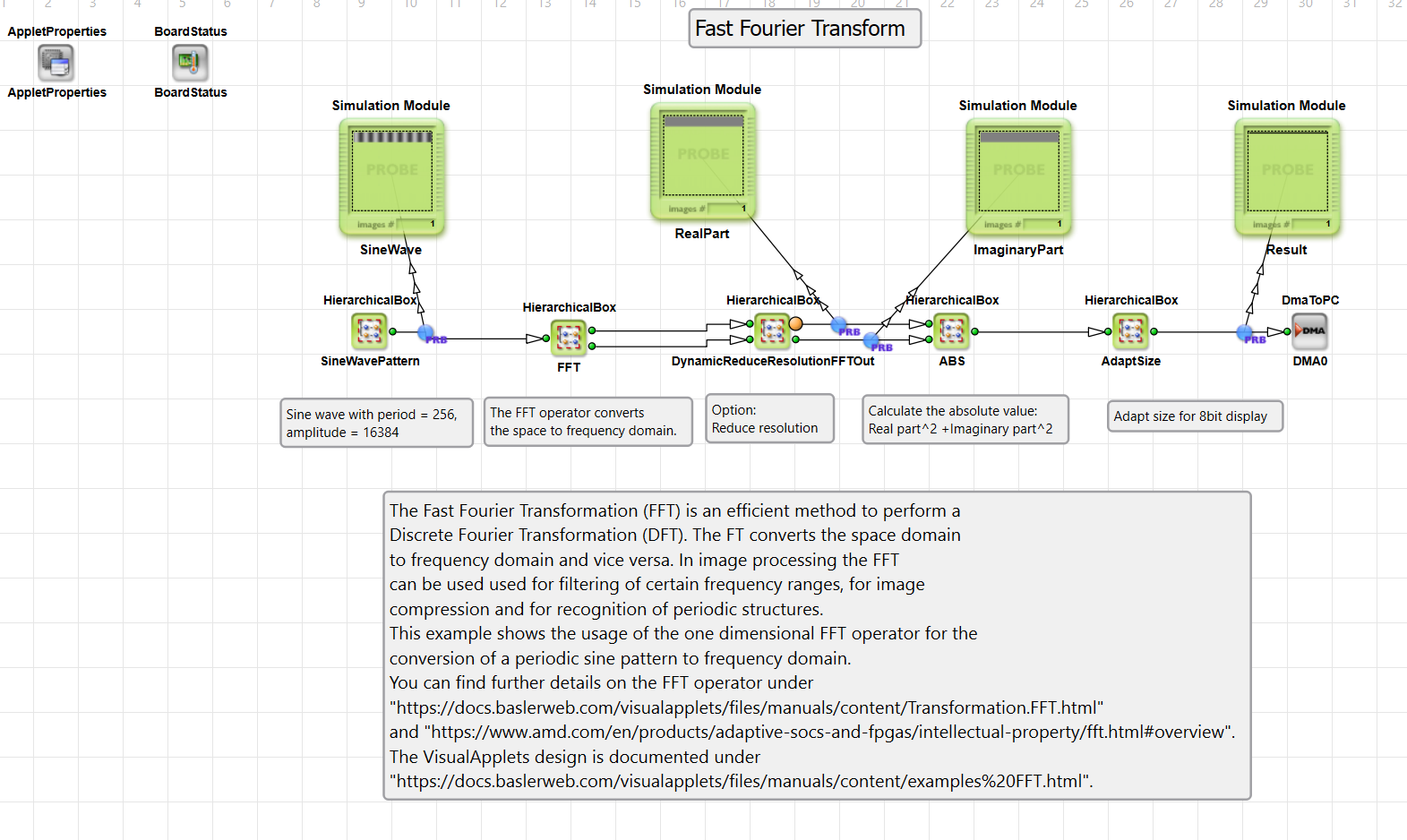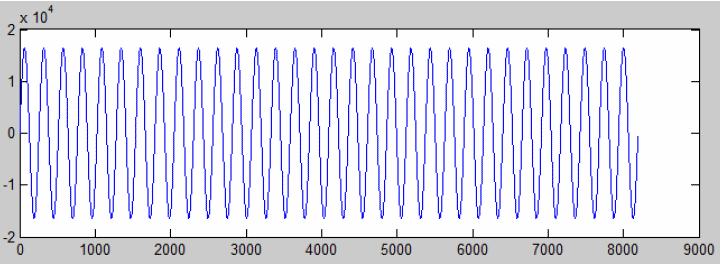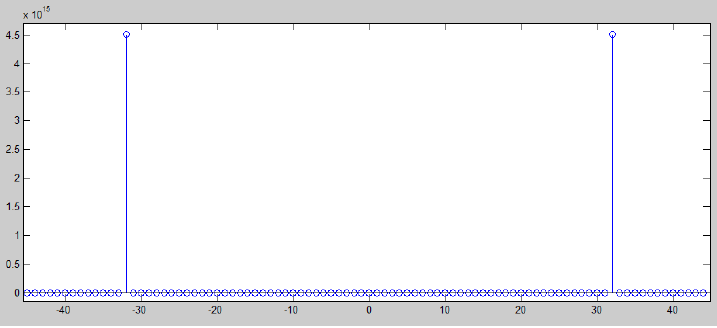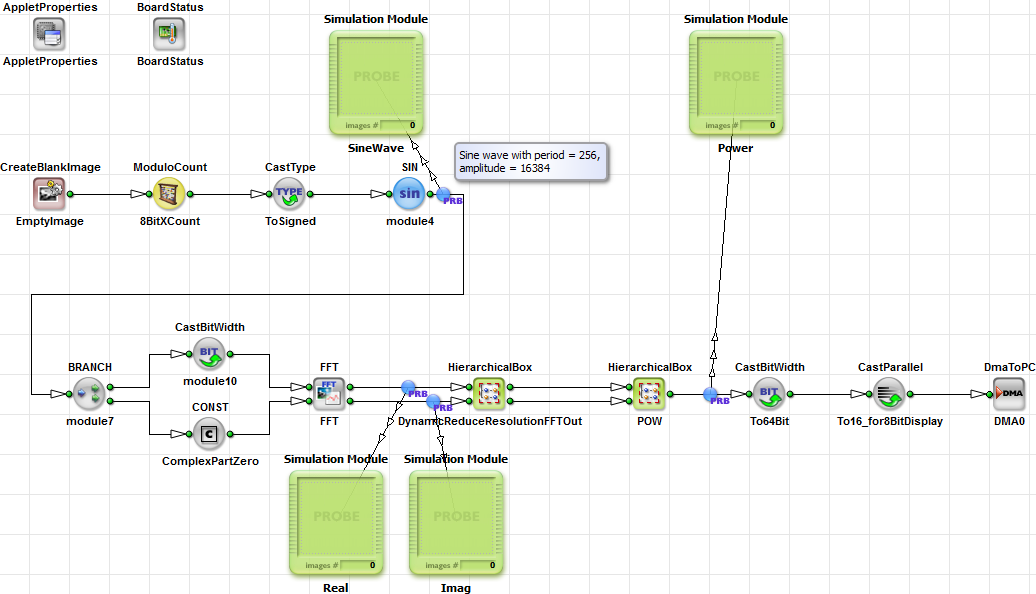### Fast Fourier transform

Brief DescriptionDefault Platform: mE5-MA-VCL or mE5-VD8-CL

Short Description

Shows the usage of operator FFT. The applet generates a sine pattern and performs the FFT. The results show the frequency part. Use the VisualApplets simulation.

The provided example will demonstrate the use of the FFT. In the example, a simple sine wave with period 256 and magnitude ± 16384 is generated.Figure 216. Sinewave

The resulting real and imaginary part are squared and added. So the output is the squared absolute value.

The result shows the expected peak at x = 32 i.e. a period of 8192 / 32 = 256. At position 32 the real part value is -43 and imaginary part is -67108738. When we compare the values with a Matlab FFT calculation we see real = 8,7e-10 and imag = 6710900. So we can see that the result is very good. There exists a small inaccuracy in the calculation only.Figure 217. FFT Result

The implementation is very simple. A sine wave input. H-Box DynamicReduceResolutionFFTOut is used to shift results and reduce their resolution. This is not required for the given sine wave.Figure 218. FFT Result